# R语言建模收入不平等：分布函数拟合及洛伦兹曲线(Lorenz curve)

## 洛伦兹曲线来源于经济学，用于描述社会收入不均衡的现象。

`````` > (income=sort(income))
  19246  23764  53237  61696 218835 ``````

``````> income/sum(income)
 0.0510
> sum(income[1:2])/sum(income)
 0.1140 ``````

`````` > plot(Lorenz(income))
> points(c(0:5)/5,c(0,cumsum(income)/sum(income)) ``````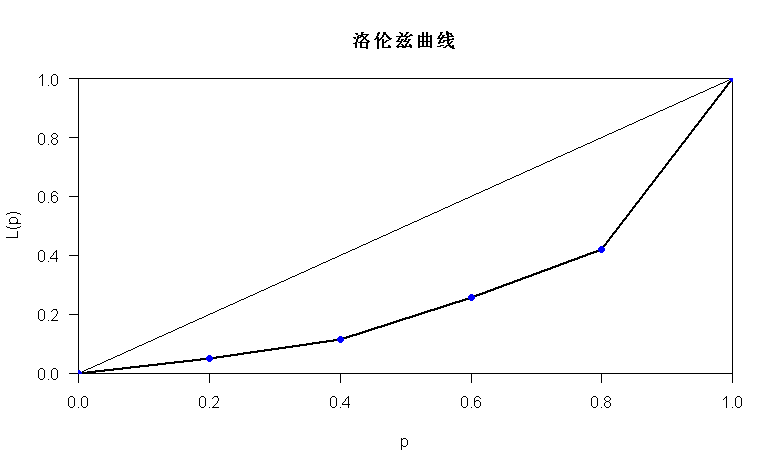``````> summary(income)
Min. 1st Qu.  Median    Mean 3rd Qu.    Max.
2191   23830   42750   77010   87430 2003000
> hist(log(income), ``````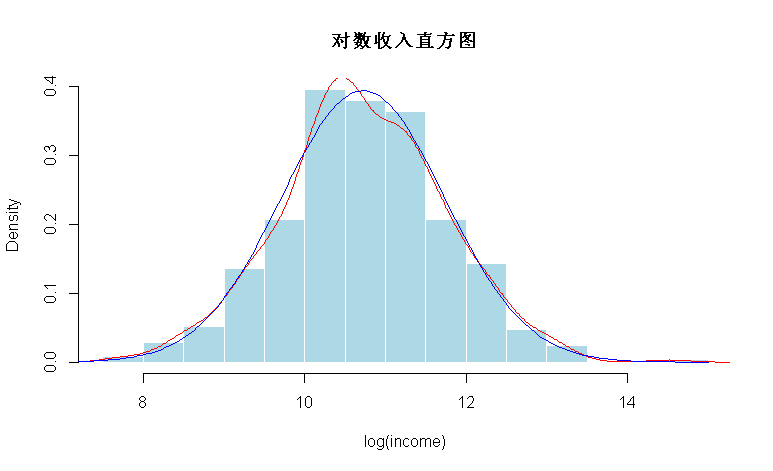``````> gini=function(x){

+ mu=mean(x)
+ g=2/(n*(n-1)*mu)*sum((1:n)*sort(x))-(n+1)/(n-1) ``````

`````` > G=boot(income,gini,1000)
> hist(G,col="light blue",border="white" ``````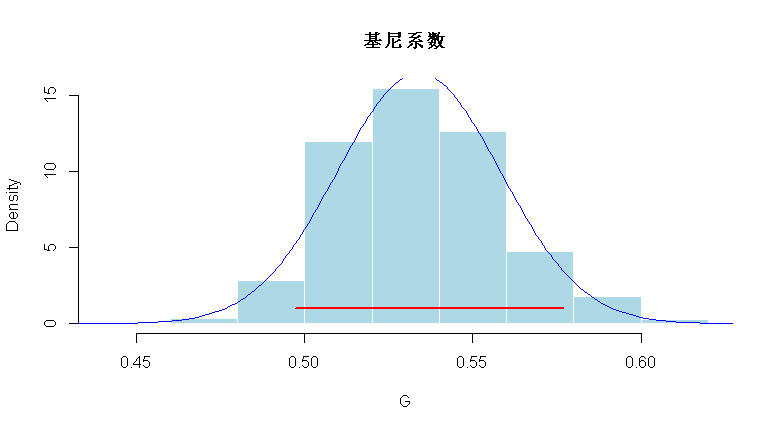`````` 5%       95%
0.4954235 0.5743917 ``````

``````> segments(quantile(G,.05),1,quantile(G,.95),1,

> lines(u,dnorm(u,mean(G),sd(G)), ``````

`` > plot(x,y) ``

# R语言用逻辑回归、决策树和随机森林对信贷数据集进行分类预测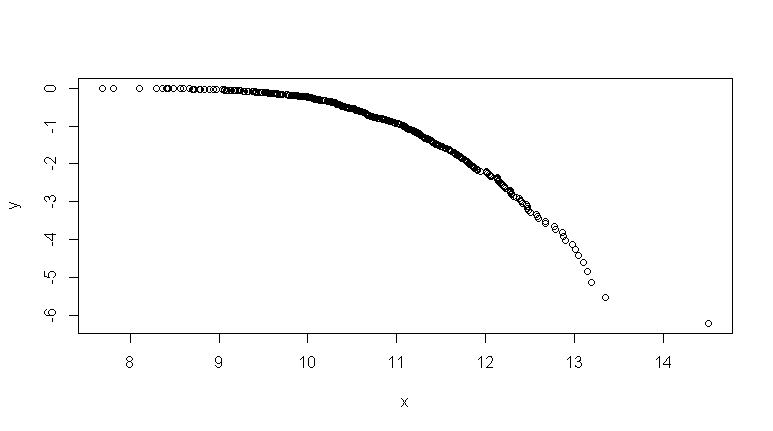`````` > lines(Lc.lognorm,param=1.5,col="red")
> lines(Lc.lognorm,param=1.2,col="red")
> lines(Lc.lognorm,param=.8,col="red") ``````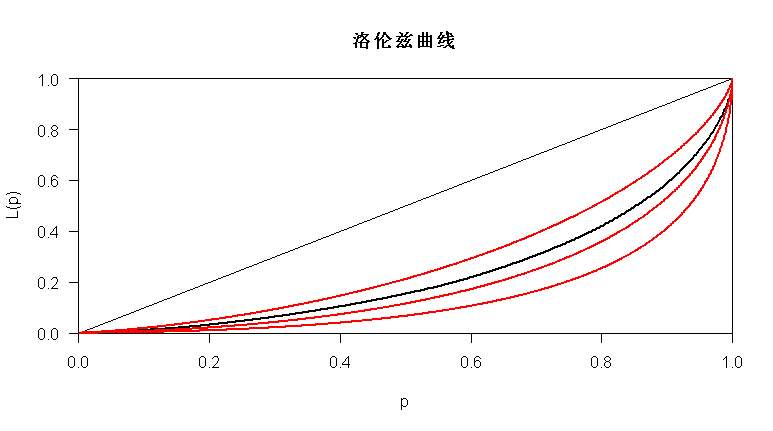### 随时关注您喜欢的主题

`` > lines(Lc.pareto,param=1.2,col="red") ``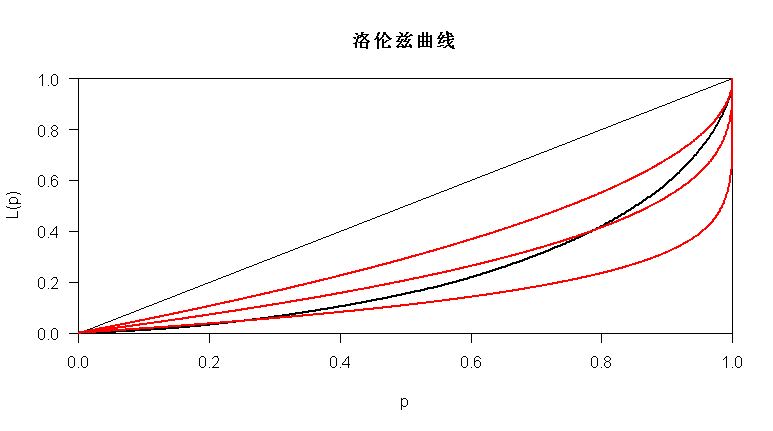`````` shape           rate
1.0812757769   0.0140404379
(0.0604530180) (0.0009868055) ``````

`````` shape           rate
1.0812757769   0.0014040438
(0.0473722529) (0.0000544185)
meanlog       sdlog
6.11747519   1.01091329
(0.04520942) (0.03196789) ``````

`````` > hist(income,breaks=seq(0,2005000,by=5000),
+ col=rgb(0,0,1,.5),border="white",
+ fit_g\$estimate)/1e2
+ fit_ln\$estimate)/1e2
> lines(u,v_g,col="red",lwd=2)
> lines(u,v_ln,col=rgb(1,0,0,.4),lwd=2) ``````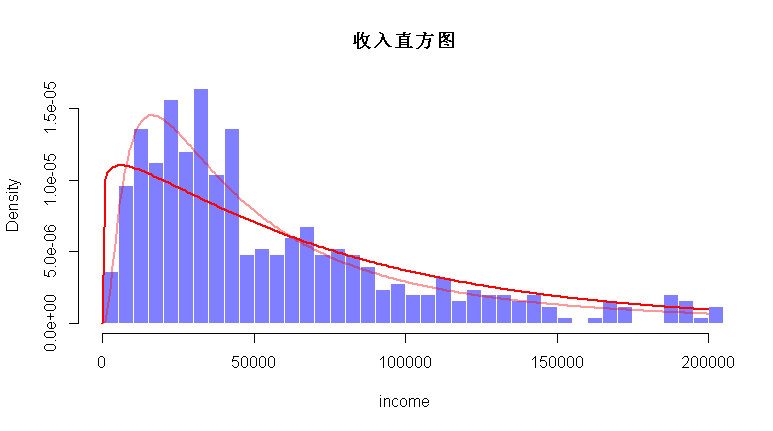# 在这里，对数正态似乎是一个不错的选择。我们还可以绘制累积分布函数

```````> plot(x,y,type="s",col="black",xlim=c(0,250000))

+ fit_g\$estimate)

+ fit_ln\$estimate)
> lines(u,v_g,col="red",lwd=2)`
``````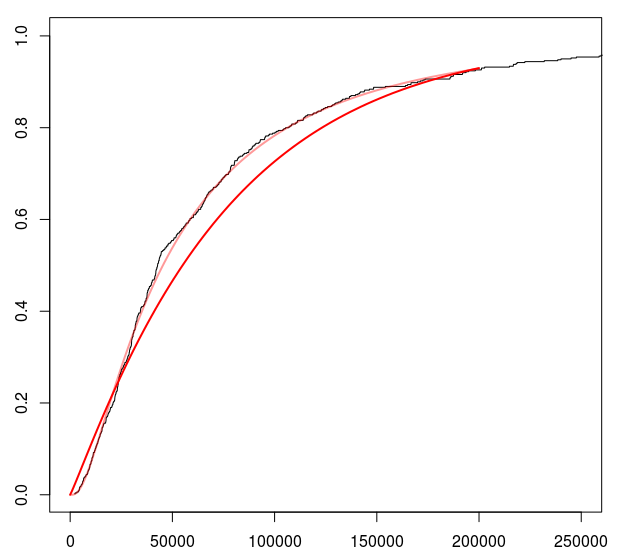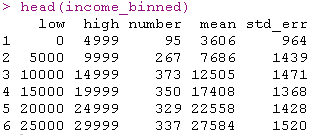``````fit(ID=rep("Data",n),

Time difference of 2.101471 secs
for LNO fit across 1 distributions ``````

# 我们可以拟合对数正态分布（有关该方法的更多详细信息，请参见 从合并收入估算不平等 的方法）

```````> y2=N/sum(N)/diff(income_binned\$low)

+ fit_LN\$parameters)
> plot(u,v,col="blue",type="l",lwd=2)
> for(i in 1:(n-1)) rect(income_binned\$low[i],0,
+ income_binned\$high[i],y2[i],col=rgb(1,0,0,.2),`
``````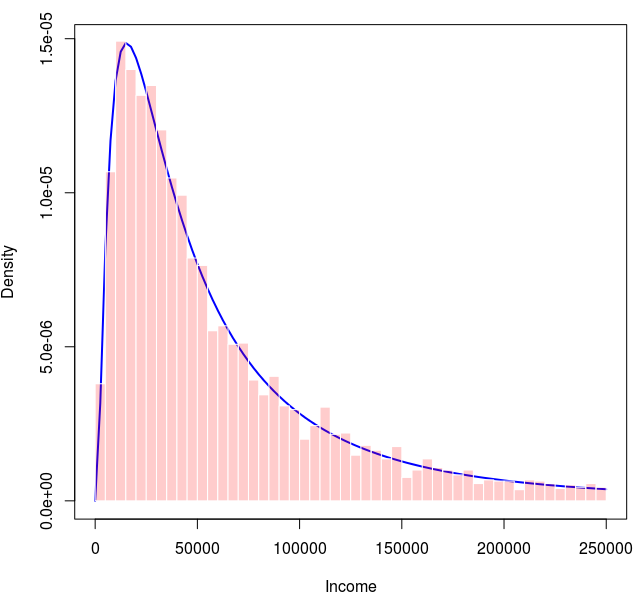``````> v <- plnorm(u,fit_LN\$parameters,
+ fit_LN\$parameters)

> for(i in 1:(n-1)) rect(income_binned\$low[i],0,

> for(i in 1:(n-1)) rect(income_binned\$low[i],
+ y1[i],income_binned\$high[i],c(0,y1)[i], ``````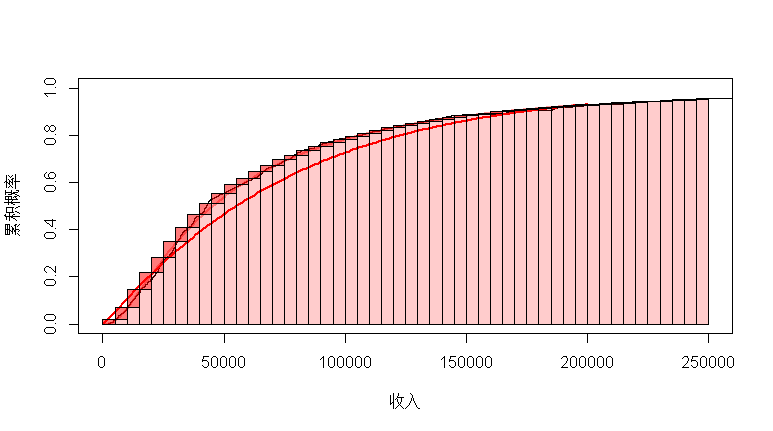``GB_family(ID=rep("Fake Data",n), ``

``> fits[,c("gini","aic","bic")] ``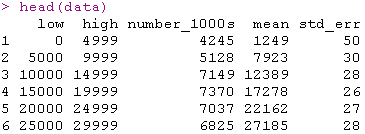`````` fit(ID=rep("US",n),

+ distribution=LNO, distName="LNO"
Time difference of 0.1855791 secs
for LNO fit across 1 distributions``````

``````> v=dlnorm(u,fit_LN\$parameters,

> plot(u,v,col="blue",type="l",lwd=2)
> for(i in 1:(n-1)) rect(data\$low[i], ``````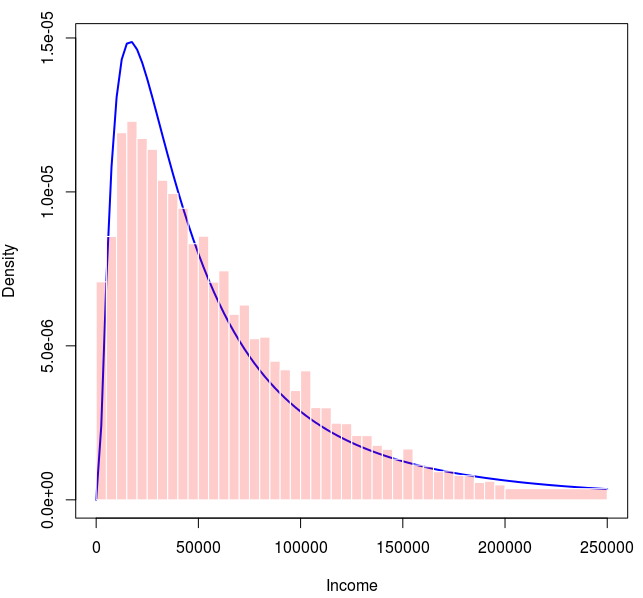``````>
GB_family(ID=rep("US",n),

+ ID_name="Country")``````

`````` gini      aic      bic
1  4.413431 825368.5 825407.3
2  4.395080 825598.8 825627.9
3  4.451881 825495.7 825524.8
4  4.480850 825881.7 825910.8
5  4.417276 825323.6 825352.7
6  4.922122 832408.2 832427.6
7  4.341036 827065.2 827084.6
8  4.318667 826112.8 826132.2
9        NA 831054.2 831073.6
10       NA       NA       NA``````

Kaizong Ye拓端研究室（TRL）的研究员。

​非常感谢您阅读本文，如需帮助请联系我们！QQ在线咨询

15121130882

0571-63341498

## 关注有关新文章的微信公众号

This will close in 0 seconds